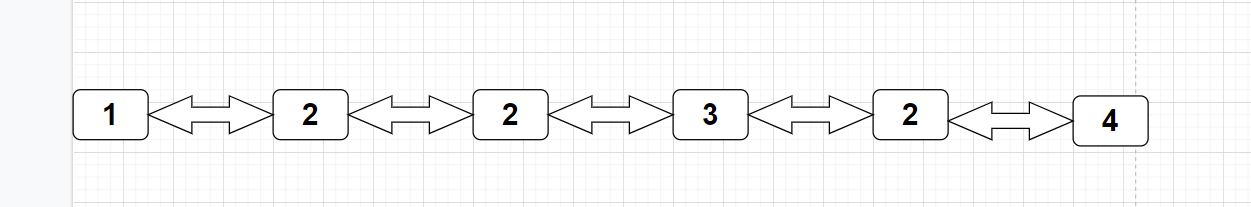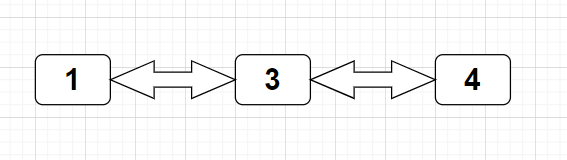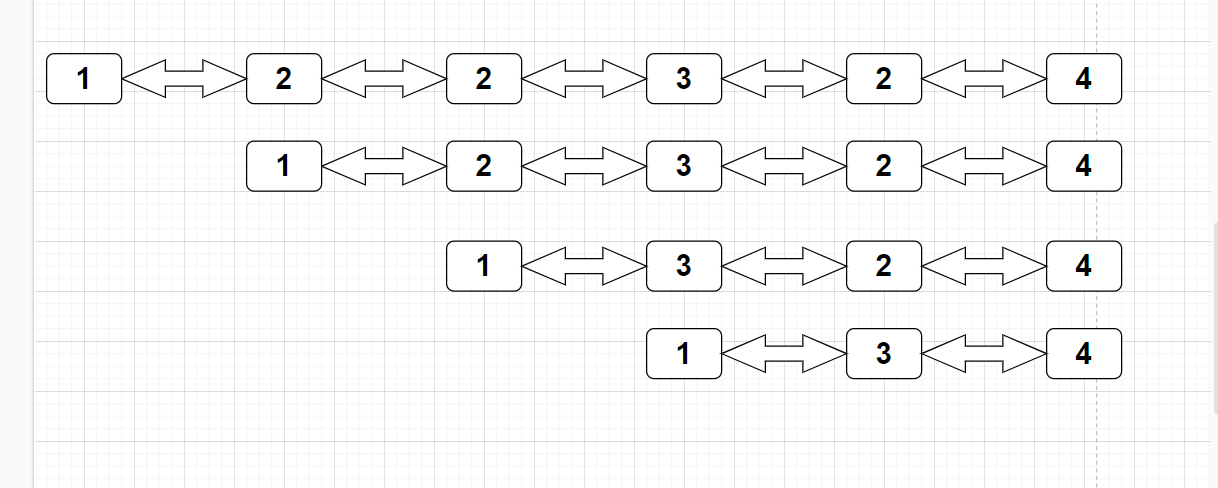New update is available. Click here to update.
Last Updated: Mar 25, 2023
Medium

# Delete all Occurrences of a given KeyAuthor Yogesh Kumar0 upvotes

## Introduction

A linked list is a linear data structure that consists of nodes. Each Node contains a data field and a pointer to the next Node. In Linked List, unlike arrays, elements are not stored at contiguous memory locations but rather at different memory locations. Linked Lists are one of the most fundamental and important data structures having a wide range of applications. Linked Lists are also important from the perspective of interviews as well.

Recommended Topic, Floyds Algorithm

## Problem Statement

In a given doubly linked list, and a key X is given, we have to delete all the occurrences of the given key X from the linked list.

Explanation of Problem Statement

In this problem statement, we have deleted all the occurrences of the given key X from the Linked List. Let’s understand the problem using the example:-

E.g.:-

Input:  1<-> 2<-> 2<-> 3<-> 2<-> 4<-> 2<-> 2      X=2Output:   1<->3<->41. As we see the above doubly linked list with the given value of X=2, we see 5 values of 2 match with the value of X, so we have to remove all the nodes with value 2 from the doubly linked list.
2. Then after removing all the occurrences of 2 from the list, we have output as      1 <-> 3 <-> 4.
3. Removing all the occurrences of given key X from the linked list means removing all the nodes from the Linked List with data equal to X.

## Approach for Solving

The approach for solving the problem is simple: we have to traverse through the Linked List from start to end and apply a check for every node. If the current node data is equal to X, we will join the current node to its next node and delete the current node.

The steps we followed to make a doubly-linked list:-

``````tail.next=newNode;
newNode.prev=tail;
tail=newNode;
tail.next=null;``````

``````tail.next=newNode;
newNode.prev=tail;
tail=newNode;
tail.next=null;``````

The steps to perform all the occurrences of X to be deleted from the linked list:-

1. If we have head==null, then nothing is there. Just return it.
2. We traverse the list one by one and check with the current.data==X if it’s found it’s a match, then call a delete function to delete the current node and link the next previous to the previous next , if current.data!=X, then we have to just shift to another node by current=current.next.

These two steps are one for creating and one for checking if it’s a match. Just delete it; otherwise, move it one step ahead.

### Code

``````public class DoublyLinkedList {
// Creating Node Class for data and storing the next and prev node
class Node{
int data;
Node prev;
Node next;
public Node(int data){
this.data=data;
}
}
Node tail=null;
// Delete the Node
// Base Condition
return null;
}
// If the deleted node is head, then we perform it
}
// Change the next, only when we have not to delete the last node
if(del.next!=null){
del.next.prev=del.prev;
}
// Change the previous, only when we have not to delete the first node
if(del.prev!=null){
del.prev.next=del.next;
}
del=null;
}
//Delete all X elements in the Doubly Linked List
public void deleteX(int X){
return;
}
Node temp_time;
while(current!=null){
if(current.data==X){
temp_time=current.next;
current=temp_time;
}
else {
current=current.next;
}
}
}
Node newNode=new Node(data);
tail.next=null;
}
else {
tail.next=newNode;
newNode.prev=tail;
tail=newNode;
tail.next=null;
}
}
// Print the Doubly Linked List.
public void printList(){
if(temp==null){
}
while(temp!=null){
System.out.print(temp.data+" ");
temp=temp.next;
}
}
public static void main(String[] args){
dl.printList();
int X=2;
dl.deleteX(X);
System.out.println();
System.out.println("Final Doubly Linked List after delete all X: ");
dl.printList();
}
}

``````

### Dry-Run

Input:  1<-> 2<-> 2<-> 3<-> 2<-> 4<-> 2<-> 2      X=2

Output:   1<->3<->4Now Let's take the above example and explain it furthermore in detail with each step working, in a dry-run manner:-

1. We choose value X=2, in which all the occurrences of node data value equal to 2 get removed.
2. We create two nodes for doing some operations so that the head of the linked list doesn't shift, now call a deleteX function to delete all the occurrences, and now declare current and temp_time in the deleteX function.
3. Now traverse the whole linked list until we have current !=null, with a condition statement over each current node if current.data!=X, then we move forward with current=current.next, if we encounter current.data==X then we have done the following steps:-
1. Now we have found the current.data==X, then we have to delete the current node.
2. For that, we have declared the temp_time node to store the address of current.next for further use.
3. Now we call a deleteNode function where we delete the Node of node data equal to X.
1. The action we do after calling the deleteNode function.
2. We perform the action we have to delete the head of the Linked List; then we have to just shift the head, as head=del.next;
3. Now we have to change the previous head node, as del.next.prev=del.prev.
4. Now we have to change the next, if we have not deleted the last node, as del.prev.next=del.next.
5. Now, at last, return the head of the linked list after applying the required modification.
4. After that present linked list gets modified.
4. Now call the print function to print the Linked List.
5. Let's perform by example:-

I=  1<-> 2<-> 2<-> 3    X=2

O= 1<->3

2. If current !=null we have a condition over current.data==X or !=X.
3. Here current =1 !=2 , then current=current.next=2.
4. Now current =2=2 , here current.data==X , then we assign temp_time=current.next=2 and call deleteNode function, after that current=temp_time=2.
1. 1<->2<->3
5. Now current=2 =2 here current.data==X , then we assign temp_time=current.next=3 and call deleteNode function, after that current=temp_time=3.
1. 1<->3
6. Now current=3!=2 here then current=current.next=null, while condition breaks as current are null, we come out from the loop and return head.
7. Now print the doubly linked list modified one is 1<-> 3 by removing all the given values of X from the Doubly Linked List.## Complexity

Now it's time to discuss the Time Complexity and Space Complexity problem for Delete all the occurrences of the given key in a doubly-linked list. As we see, we are forming the linked list, which generally takes O(constant) time for each node creating at every time and space it occupies as a doubly-linked list for storing the next and previous address of the node.

Now, let’s talk about the Time Complexity after completion of the linked list, Time Complexity to delete all the occurrences of the X in the Linked List, as we see in the code. We are iterating from start to end of the list one by one to check current.data==X, which will happen in O(Length of Linked List==L), i.e., O(L) where L is the length of the doubly linked list.

If we talk about the Space Complexity, as we are just maintaining the created doubly linked list, we are not going to make duplicate, or we have not moved anywhere node by creating another node and assigning to create another linked list, we are just creating the variable Node for pointing the same Doubly Linked List, So the Space Complexity of the problem is O(1). We are not using any extra space.

Check out this problem - Find Duplicate In Array

### What are Time Complexity and Space Complexity for deleting all X elements in a list?

Time Complexity is O(N) and Space Complexity is O(1).

### What is the operation performed on the Doubly Linked List?

All the standard data structure operations we can perform over it. Like: Insertion, Deletion, etc.

## Conclusion

In this blog, we learn about the Doubly Linked List, how to create the DLL, how the delete operation performs in the Doubly Linked List, and how the next and prev of the node maintain the address of the next and previous the current node. We also discussed the time and space complexity with a dry run over an example.

Apart from this, you can practice a wide range of coding questions commonly asked in interviews in CodeStudio. Along with coding questions, we can also find the interview experience of scholars working in renowned product-based companies here.

If you want to know about the implementation of a doubly-linked list from the scratch then you can check the blog Introduction and Implementation of Doubly Linked ListsGo on top